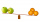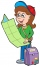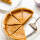# Fraction calculator

The calculator performs basic and advanced operations with fractions, expressions with fractions combined with integers, decimals, and mixed numbers. It also shows detailed step-by-step information about the fraction calculation procedure. Solve problems with two, three, or more fractions and numbers in one expression.

## Result:

### 12/3 * 31/2 = 35/6 = 5 5/6 ≅ 5.8333333

Spelled result in words is thirty-five sixths (or five and five sixths).

### How do you solve fractions step by step?

1. Conversion a mixed number 1 2/3 to a improper fraction: 1 2/3 = 1 2/3 = 1 · 3 + 2/3 = 3 + 2/3 = 5/3

To find a new numerator:
a) Multiply the whole number 1 by the denominator 3. Whole number 1 equally 1 * 3/3 = 3/3
b) Add the answer from previous step 3 to the numerator 2. New numerator is 3 + 2 = 5
c) Write a previous answer (new numerator 5) over the denominator 3.

One and two thirds is five thirds
2. Conversion a mixed number 3 1/2 to a improper fraction: 3 1/2 = 3 1/2 = 3 · 2 + 1/2 = 6 + 1/2 = 7/2

To find a new numerator:
a) Multiply the whole number 3 by the denominator 2. Whole number 3 equally 3 * 2/2 = 6/2
b) Add the answer from previous step 6 to the numerator 1. New numerator is 6 + 1 = 7
c) Write a previous answer (new numerator 7) over the denominator 2.

Three and one half is seven halfs
3. Multiple: 5/3 * 7/2 = 5 · 7/3 · 2 = 35/6
Multiply both numerators and denominators. Result fraction keep to lowest possible denominator GCD(35, 6) = 1. In the following intermediate step, the fraction result cannot be further simplified by canceling.
In other words - five thirds multiplied by seven halfs = thirty-five sixths.

#### Rules for expressions with fractions:

Fractions - simply use a forward slash between the numerator and denominator, i.e., for five-hundredths, enter 5/100. If you are using mixed numbers, be sure to leave a single space between the whole and fraction part.
The slash separates the numerator (number above a fraction line) and denominator (number below).

Mixed numerals (mixed fractions or mixed numbers) write as integer separated by one space and fraction i.e., 1 2/3 (having the same sign). An example of a negative mixed fraction: -5 1/2.
Because slash is both signs for fraction line and division, we recommended use colon (:) as the operator of division fractions i.e., 1/2 : 3.

Decimals (decimal numbers) enter with a decimal point . and they are automatically converted to fractions - i.e. 1.45.

The colon : and slash / is the symbol of division. Can be used to divide mixed numbers 1 2/3 : 4 3/8 or can be used for write complex fractions i.e. 1/2 : 1/3.
An asterisk * or × is the symbol for multiplication.
Plus + is addition, minus sign - is subtraction and ()[] is mathematical parentheses.
The exponentiation/power symbol is ^ - for example: (7/8-4/5)^2 = (7/8-4/5)2

#### Examples:

subtracting fractions: 2/3 - 1/2
multiplying fractions: 7/8 * 3/9
dividing Fractions: 1/2 : 3/4
exponentiation of fraction: 3/5^3
fractional exponents: 16 ^ 1/2
adding fractions and mixed numbers: 8/5 + 6 2/7
dividing integer and fraction: 5 ÷ 1/2
complex fractions: 5/8 : 2 2/3
decimal to fraction: 0.625
Fraction to Decimal: 1/4
Fraction to Percent: 1/8 %
comparing fractions: 1/4 2/3
multiplying a fraction by a whole number: 6 * 3/4
square root of a fraction: sqrt(1/16)
reducing or simplifying the fraction (simplification) - dividing the numerator and denominator of a fraction by the same non-zero number - equivalent fraction: 4/22
expression with brackets: 1/3 * (1/2 - 3 3/8)
compound fraction: 3/4 of 5/7
fractions multiple: 2/3 of 3/5
divide to find the quotient: 3/5 ÷ 2/3

The calculator follows well-known rules for order of operations. The most common mnemonics for remembering this order of operations are:
PEMDAS - Parentheses, Exponents, Multiplication, Division, Addition, Subtraction.
BEDMAS - Brackets, Exponents, Division, Multiplication, Addition, Subtraction
BODMAS - Brackets, Of or Order, Division, Multiplication, Addition, Subtraction.
GEMDAS - Grouping Symbols - brackets (){}, Exponents, Multiplication, Division, Addition, Subtraction.
Be careful, always do multiplication and division before addition and subtraction. Some operators (+ and -) and (* and /) has the same priority and then must evaluate from left to right.

## Fractions in word problems:

• DividendsThe three friends divided the win by the invested money. Karlos got three-eighths, John 320 permille, and the rest got Martin. Who got the most and which the least?
• TorqueTorque and Mari each multiplied 1/8 inch times 5/8 inch. Tartaric 5/8 squares point inches. And Marie got 5/64 squared thought inches tall. Which student found a corrupt area?
• Ten fractionsWrite ten fractions between 1/3 and 2/3
• Comparing and sortingArrange in descending order this fractions: 2/7, 7/10 & 1/2
• Equivalent fractionsAre these two fractions equivalent -4/9 and 11/15?
• Turtles 2A box turtle hibernates in the sand at 11 5/8. A spotted turtle hibernates at 11 16/25 feet. Which turtle is deeper? Write answer as number 1 or 2.
• Giraffes to monkeysThe ratio of the number of giraffes to the number of monkeys in a zoo is 2 to 5. Which statement about the giraffes and monkeys could be true? A. For every 10 monkeys in the zoo, there are 4 giraffes. B. For every  giraffe in the zoo, there are 3 monkeys.
• Equivalent expressionsA coach took his team out for pizza after their last game. There were 14 players, so they had to sit in smaller groups at different tables. Six players sat at one table and got 4 small pizzas to share equally. The other players sat at the different table
• Sort fractionsWhich of the following fractions is the largest? 29/36 5/6 7/9 3/4
• LukeLuke, Seth, and Anja have empty glasses. Mr. Gabel pours 3/6 cup of orange juice in Seth's glass. Then he pouts 1/6 cup of orange juice in Luke's glass and 2/6 cup of orange juice in Anja's glass. Who gets the MOST orange juice?
• Leo hikedLeo hiked 6/7 of a kilometer. Jericho hiked 2/3 kilometer. Who covered a longer distance? How much longer?
• SandySandy, John and Marg baked pies for the Bake Sale. Sandy cut his pies into 6ths, John but his into 8ths, and Marg cut hers into quarters. Sandy sold 11/6, John sold  1 3/8 pies, and Marg sold 9/4 pies. Who sold the most pies? Who sold the fewest?
• Pumpkin pieHave some pumpkin pie. One half of the pie is cut into 4 equal slices, the other half is cut into 3 equal slices. After eating one of the larger slices (on the 3 piece side), I wonder if I will eat more if I have one more from the 3 side, or two from the